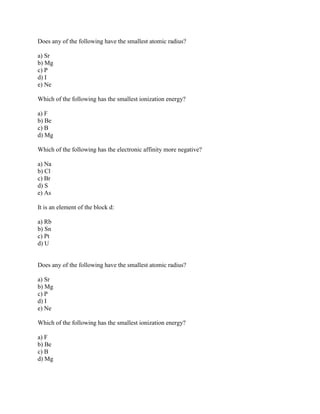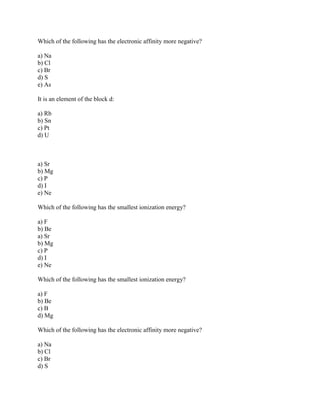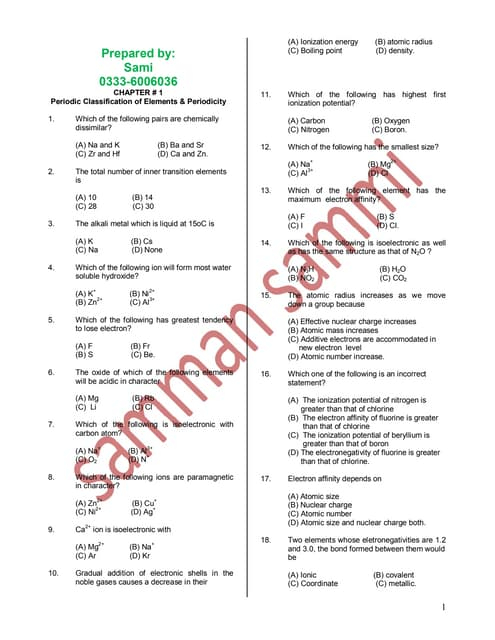PublicitéProchain SlideShareChemstry 2nd book
Chargement dans ... 3
1 sur 3
Publicité

### Does any of the following have the smallest atomic radius- a) Sr b) M.docx

1. Does any of the following have the smallest atomic radius? a) Sr b) Mg c) P d) I e) Ne Which of the following has the smallest ionization energy? a) F b) Be c) B d) Mg Which of the following has the electronic affinity more negative? a) Na b) Cl c) Br d) S e) As It is an element of the block d: a) Rb b) Sn c) Pt d) U Does any of the following have the smallest atomic radius? a) Sr b) Mg c) P d) I e) Ne Which of the following has the smallest ionization energy? a) F b) Be c) B d) Mg
2. Which of the following has the electronic affinity more negative? a) Na b) Cl c) Br d) S e) As It is an element of the block d: a) Rb b) Sn c) Pt d) U a) Sr b) Mg c) P d) I e) Ne Which of the following has the smallest ionization energy? a) F b) Be a) Sr b) Mg c) P d) I e) Ne Which of the following has the smallest ionization energy? a) F b) Be c) B d) Mg Which of the following has the electronic affinity more negative? a) Na b) Cl c) Br d) S
3. e) As It is an element of the block d: a) Rb b) Sn c) Pt d) U Solution 1. Ne has smallest atomic radius. 2. The smallest ionization is for Mg( Because it has large atomic size compared to remaining so that we can take out one electron easily. The energy is called ionization energy) 3. Cl has more electronegativity value. Because it can easily takes electron and become negative. 4. Pt is D block element.
Publicité